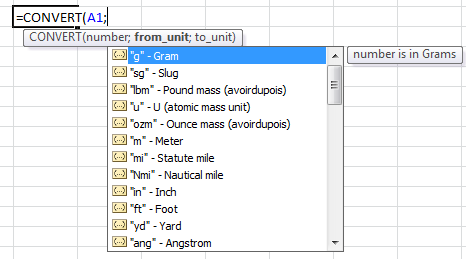# Unit Conversion In Excel

Excel has a built-in unit converter. Not surprising isn’t it? Unit conversion is excel is done with CONVERT() Function. Here is how it works…Convert fuction’s syntax is:

=CONVERT(number, from unit, to unit)

Lets see some examples:

Assume, Cell A1 holds value 10.

Km to m : =CONVERT(A1;”km”;”m”) will result 10.000

Kg to g : =CONVERT(A1;”kg”;”g”) will result 10.000

Year to day : =CONVERT(A1;”yr”;”day”) will result 3.652,5

In order to use convert function, you need to input units for conversions. This means you are expected to know short names of units that are recognized by excel.  As you write your formula, excel will suggest some units for easy use:Here is also a full list of units (taken from excel help).

 Weight and mass From_unit or to_unit Gram “g” Slug “sg” Pound mass (avoirdupois) “lbm” U (atomic mass unit) “u” Ounce mass (avoirdupois) “ozm” Distance From_unit or to_unit Meter “m” Statute mile “mi” Nautical mile “Nmi” Inch “in” Foot “ft” Yard “yd” Angstrom “ang” Pica “pica” Time From_unit or to_unit Year “yr” Day “day” Hour “hr” Minute “mn” Second “sec” Pressure From_unit or to_unit Pascal “Pa” (or “p”) Atmosphere “atm” (or “at”) mm of Mercury “mmHg” Force From_unit or to_unit Newton “N” Dyne “dyn” (or “dy”) Pound force “lbf” Energy From_unit or to_unit Joule “J” Erg “e” Thermodynamic calorie “c” IT calorie “cal” Electron volt “eV” (or “ev”) Horsepower-hour “HPh” (or “hh”) Watt-hour “Wh” (or “wh”) Foot-pound “flb” BTU “BTU” (or “btu”) Power From_unit or to_unit Horsepower “HP” (or “h”) Watt “W” (or “w”) Magnetism From_unit or to_unit Tesla “T” Gauss “ga” Temperature From_unit or to_unit Degree Celsius “C” (or “cel”) Degree Fahrenheit “F” (or “fah”) Kelvin “K” (or “kel”) Liquid measure From_unit or to_unit Teaspoon “tsp” Tablespoon “tbs” Fluid ounce “oz” Cup “cup” U.S. pint “pt” (or “us_pt”) U.K. pint “uk_pt” Quart “qt” Gallon “gal” Liter “l” (or “lt”)

The following abbreviated unit prefixes can be prepended to any metric from_unit or to_unit.

 Prefix Multiplier Abbreviation exa 1.00E+18 “E” peta 1.00E+15 “P” tera 1.00E+12 “T” giga 1.00E+09 “G” mega 1.00E+06 “M” kilo 1.00E+03 “k” hecto 1.00E+02 “h” dekao 1.00E+01 “e” deci 1.00E-01 “d” centi 1.00E-02 “c” milli 1.00E-03 “m” micro 1.00E-06 “u” nano 1.00E-09 “n” pico 1.00E-12 “p” femto 1.00E-15 “f” atto 1.00E-18 “a”

#### You may also like...

This site uses Akismet to reduce spam. Learn how your comment data is processed.